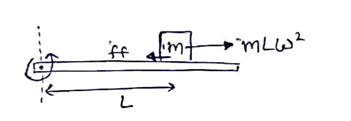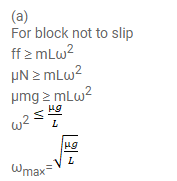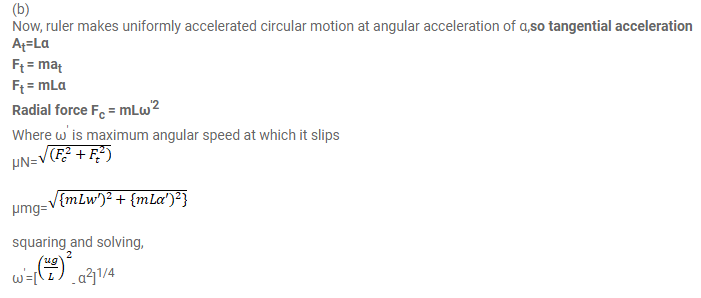Deepak Scored 45->99%ile with Bounce Back Crack Course. You can do it too!

# A block of mass m is kept on a horizontal ruler.

Question:

A block of mass $m$ is kept on a horizontal ruler. The friction coefficient between the ruler and the block is g. The ruler is fixed at one end and the block is at a distance $L$ from the fixed end. The ruler is rotated about the fixed end in the horizontal plane through the fixed end.

(a) What can the maximum angular speed be for which the block does not slip?

(b) If the angular speed of the ruler is uniformly increased from zero at an angular acceleration a, at what angular speed will the block slip?

Solution: